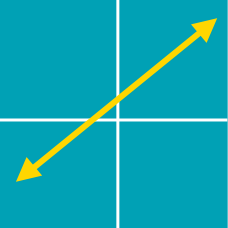Algebra

# Finding Points on a Line

The point $(12, k)$ lies on the line $y = 2x + 7$. What is the value of $k$?

If $P=(a, 3)$ is a point on the line $x+y-11=0,$ what is the value of $a$?

If the graph of $y=7x-20$ passes through two points $A=(-1,p)$ and $B=(q,14),$ what is the value of $p+28q?$

How many points are there on the line $x+2y=9$ such that the $x$- and $y$-coordinates are both non-negative integers?

If $P=(5, a)$ is a point on the line $3x+y-22=0,$ what is the value of $a$?

×